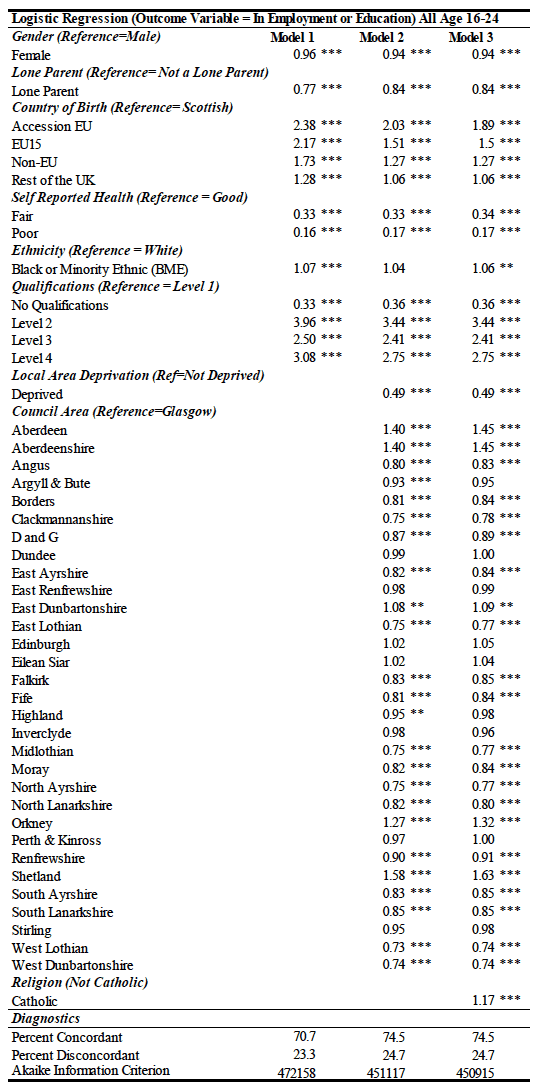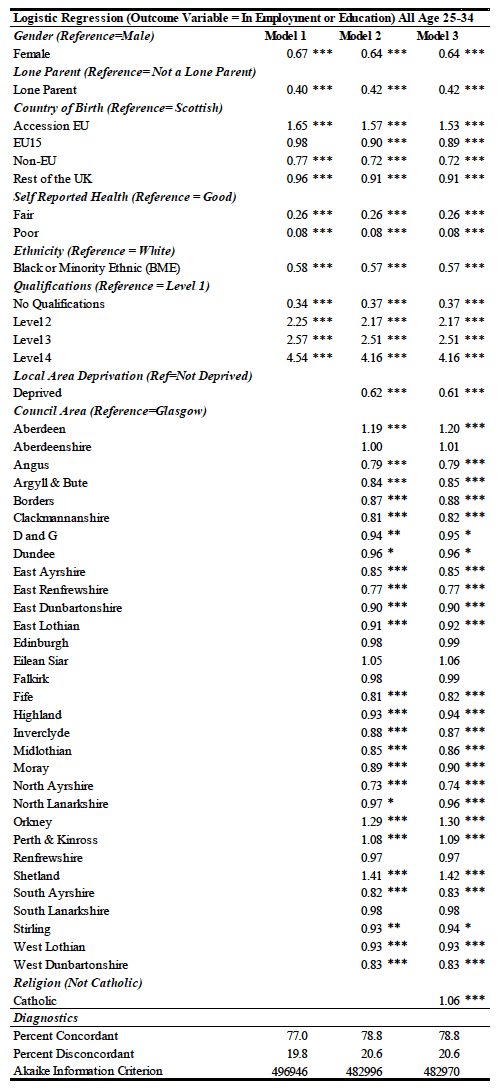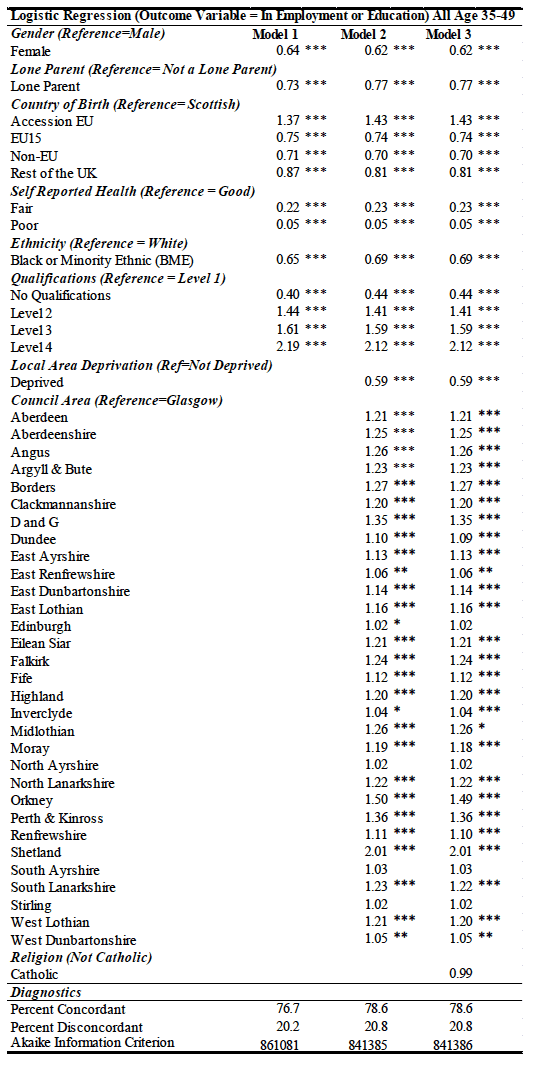# An Examination of the Evidence on Sectarianism in Scotland: 2015 Update

A summary of the evidence on sectarianism in Scotland

### Further tables

Appendix - Detailed Models

Table 17: Logistic Regression for 16-24 year-olds (*** refers to a p-value less than 0.01, ** a p-value less than 0.05, and * a p-value less than 0.1)Table 18: Logistic Regression for 25-34 year-olds (*** refers to a p-value less than 0.01, ** a p-value less than 0.05, and * a p-value less than 0.1)Table 19: Logistic Regression for 35-49 year-olds (*** refers to a p-value less than 0.01, ** a p-value less than 0.05, and * a p-value less than 0.1)### Contact

Email: Ben Cavanagh